# Arf-invariant

(diff) ← Older revision | Latest revision (diff) | Newer revision → (diff)

invariant of Arf

An invariant of a quadratic form modulo 2, given on an integral lattice endowed with a bilinear skew-symmetric form. Let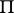be an integral lattice of dimension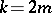and letbe a form for which. There exists bases, called symplectic bases, in which the matrix of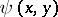reduces to block-diagonal form: The diagonal contains the blocks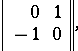i.e.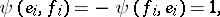while the other entries are zero.

Suppose that a mappingis given onsuch that(a "quadratic form modulo 2" ). The expressionis then called an Arf-invariant . If this expression equals zero, then there is a symplectic basis on all elements of whichvanishes; if this expression equals one, then there is a symplectic basis on all elements of which, exceptand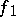, the form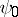vanishes, while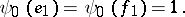How to Cite This Entry:
Arf-invariant. Encyclopedia of Mathematics. URL: http://encyclopediaofmath.org/index.php?title=Arf-invariant&oldid=12338
This article was adapted from an original article by A.V. Chernavskii (originator), which appeared in Encyclopedia of Mathematics - ISBN 1402006098. See original article